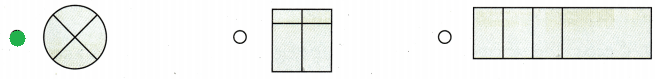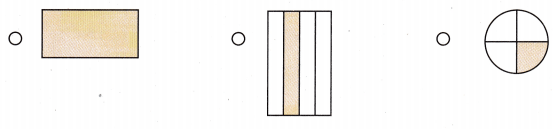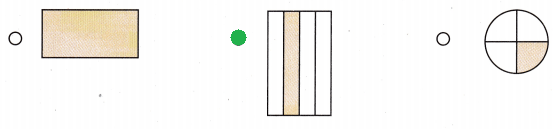Refer to our Texas Go Math Grade 1 Answer Key Pdf to score good marks in the exams. Test yourself by practicing the problems from Texas Go Math Grade 1 Module 16 Assessment Answer Key.

Vocabulary

Draw a line to show halves. (p.578)

Question 1.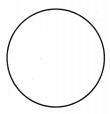Explanation:
To divide a circle into halves draw a line in the center it will give you two equal halves of a circle

Question 2.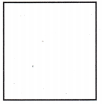Explanation:
To divide a Square into halves draw a line it will give you two equal halves of a square

Question 3.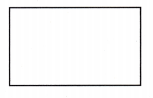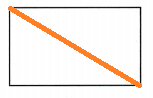Explanation:
To divide a rectangle into halves draw a line it will give you two equal halves of a rectangle

Draw lines to show fourths.

Question 4.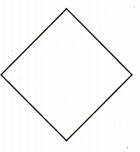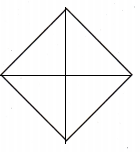Explanation:
To divide a square into forth  draw a two lines so that  it will give you four equal halves of a square

Question 5.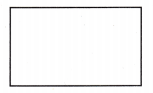Explanation:
To divide a Rectangle into forth  draw a two lines so that  it will give you four equal halves of a rectangle

Question 6.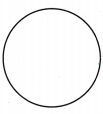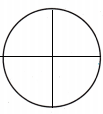Explanation:
To divide a circle into forth  draw a two lines so that  it will give you four equal halves of a circle

Concepts and Skills

Question 7.
Color the shapes that show unequal shares. (TEKS 1.6.G, 1.6.H )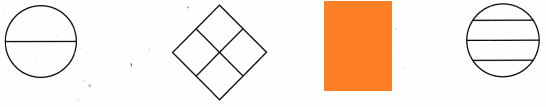Explanation:
In the above diagram all the three are divided into equal but the rectangle is not divided into equal parts

Question 8.
Color the shapes that show equal shares. (TEKS 1.6.G, 1.6.H )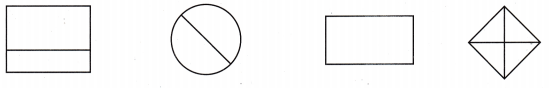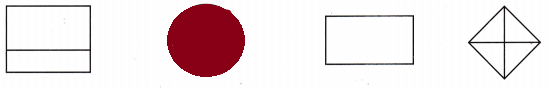Explanation:
In the above all the unequally divided except the circle its equally divided

Question 9
Which shows halves? (TEKS 1.6.G, 1.6.H)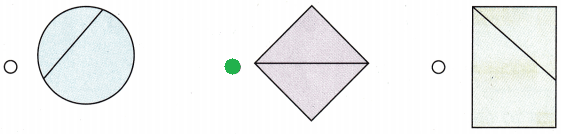Explanation:
The shape that show halves is the second shape as a is divided into two parts and is also divided into equal parts

Question 10.
Which shape shows unequal parts? (TEKS 1.6.G, 1.6.H)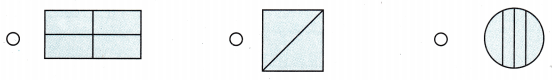Explanation:
The shape shows unequal parts is the circle as its divided into four parts but is not divided equally.

Question 11.
Which shading shows half of a square? (TEKS 1.6.G, 1.6.H)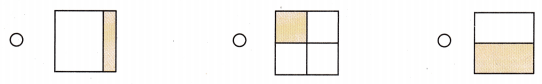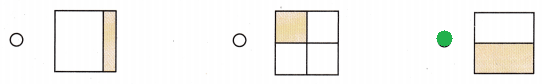Explanation:
The shading that shows half of a square the third one as its equally divided

Question 12.
Which shape shows fourths? (TEKS 1.6.G, 1.6.H)